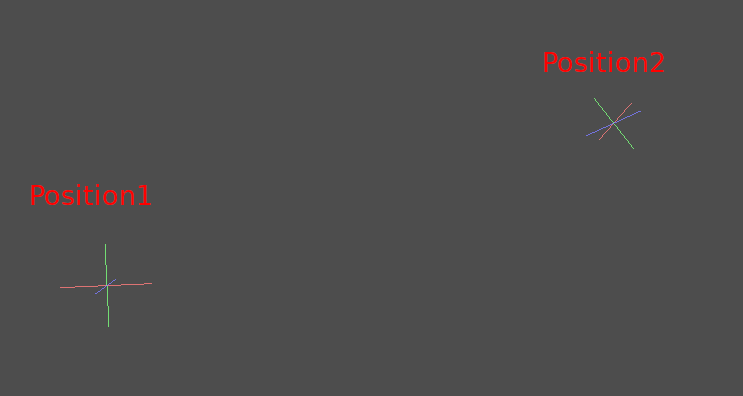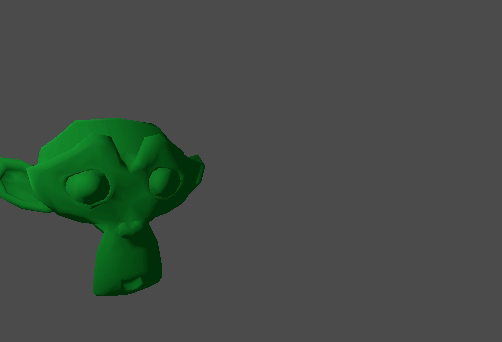# 插值¶

```interpolation = A * (1 - t) + B * t
```

```interpolation = A + (B - A) * t
```

## Vector interpolation¶

```func _physics_process(delta):
t += delta * 0.4

\$Sprite.position = \$A.position.linear_interpolate(\$B.position, t)
```

It will produce the following motion:## Transform interpolation¶Using the following pseudocode:

```var t = 0.0

func _physics_process(delta):
t += delta

\$Monkey.transform = \$Position1.transform.interpolate_with(\$Position2.transform, t)
```

And again, it will produce the following motion:## Smoothing motion¶

```const FOLLOW_SPEED = 4.0

func _physics_process(delta):
var mouse_pos = get_local_mouse_position()

\$Sprite.position = \$Sprite.position.linear_interpolate(mouse_pos, delta * FOLLOW_SPEED)
```

Here is how it looks: